# Usage tutorial

GATree is a decision tree builder that is based on Genetic Algorithms (GAs). The idea behind it is rather simple but powerful. Instead of using statistic metrics that are biased towards specific trees we use a more flexible, global metric of tree quality that try to optimize accuracy and size. GATree offers some unique features not to be found in any other tree inducers while at the same time it can produce better results for many difficult problems.

A few unique characteristics of GATree are as follows:

- The user can control the characteristics of the output (More Accurate vs Smaller Trees)
- There is no upper bound on its results given that we can provide the system with unlimited processing power and time
- The system evolves complete solutions to the problem. We can stop evolution whenever the results are satisfactory
- The system evolves a set of possible solutions (e.g, decision trees) that closely match the input data. This gives us alternative hypotheses for the same data

In this small tutorial we will comment on a few screenshots from the program’s interface that uncover its basic functionality. We will also present a simple step-by-step example with instructions on how to use and decode the program’s output effectively.

Evolve Tab

The main screen of the program (Figure 1) allows us to select an active training dataset and evolve the decision tree. In the main program’s window we can watch the best decision tree as it evolves through time. The right panel includes info about the current status of the evolution process.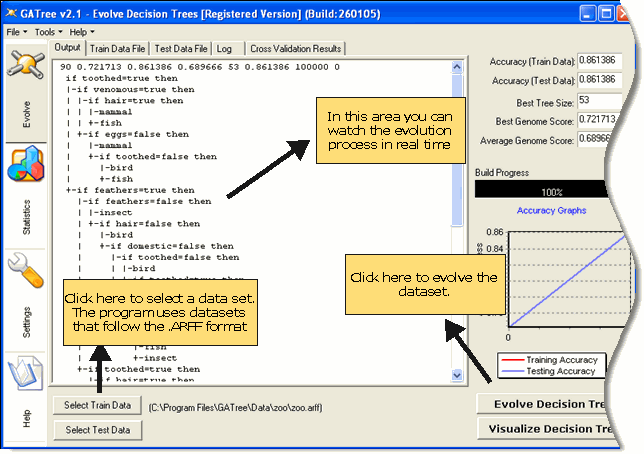Figure 1: The main evolution tab

By pressing the Visualize-Decision-Tree button we can visualize and traverse the final decision tree (Figure 2)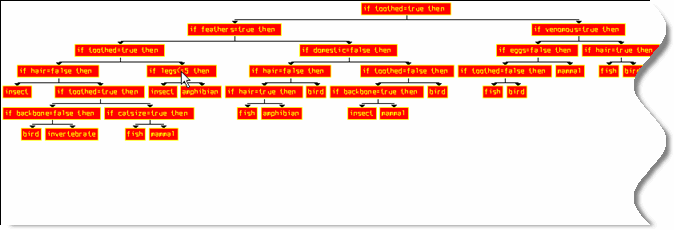Figure 2: Decision tree visualization

Statistics Tab

The statistics tab (Figure 3) provides several graphs of the evolution process. Those graphs allows us to follow in real time the evolution process and discover potential problems and trends. As an example, when the Average Fitness of the population tend to be equal to the Fitness of the best Genome then there is little room for further improvements. A solution here could be to try with more generations or bigger population size.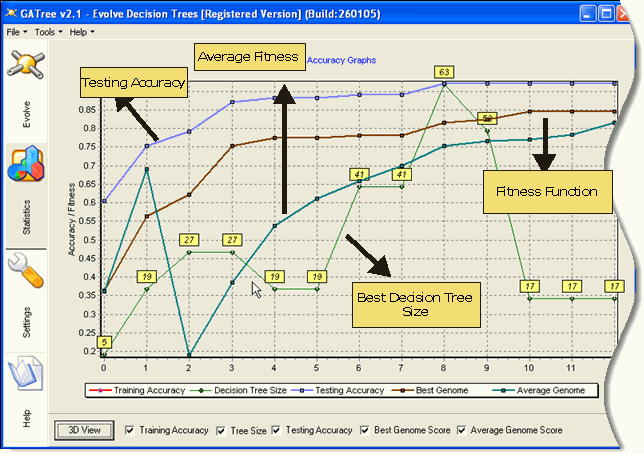Figure 3: The statistics tab

Settings Tab

The settings tab (Figure 4) allows us to control every aspect of the evolution process. There are two types of settings; Basic settings and Advanced settings depending on their usefulness and complexity. Below you can find an explanation of the offered options.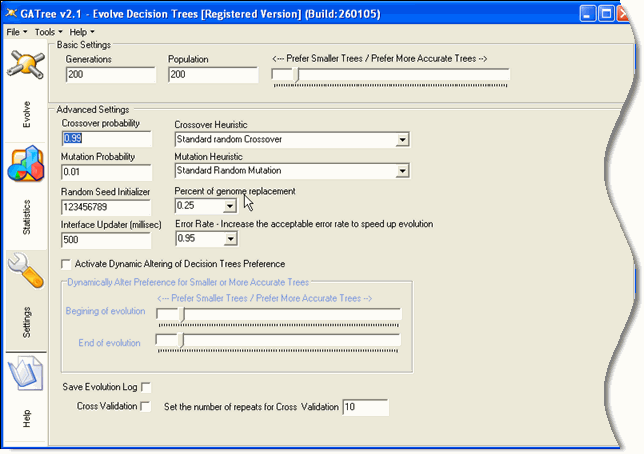Figure 4: The settings tab

Generations/Population:
Those two options control the number of generation and the initial population of random decision trees. They effectively control the total time of evolution but also the quality of the output.

Small/Accurate Trees slider:
A unique characteristic of the genetic evolution is that we can decide about the generic characteristics of the output.The slider here allows us to decide whether we would like to search in a bigger or smaller solution space. If is set to the left it enforces a penalty to all big trees that excludes them from next generations best population. You can also dynamically alter this preference by setting if for the beggining and ending of evolution.This way the program will slowly move during the evolution for a preference for a big tree to a preference for a smaller tree or vice versa (advanced settings).

Crossover / Mutation Probability:
From here we control the genetic characteristics of the evolution. Crossover probability refers to the probability that a random subtree will be replaced with another sub-tree. Mutation probability refers to the probability for a node to be randomly altered to include a new value.

Crossover / Mutation Heuristic:
Those two options allow us to select among several different versions of the standard crossover/mutation that prefer nodes or good sub-trees. The idea here is that those alterations may improve the speed and accuracy of evolution.

Random Seed Initializer:
This is a random sequence generator. If we keep the initializer to viagra cialis the same number then we can expect the same decision tree for the same settings. Otherwise, the results will tend to be the same but due to the fuzziness of the genetic evolution, exact same results are not guaranteed.

Percent of genome replacement:
This parameter control the number of bad trees that will be replaced with new ones between generations.

Error rate:
This is another speed up parameter. When the error rate of a decision tree surpass a limit we avoid classifying more testing instances to preserve resources.

Interface Updater:
It controls how often the interface will update the statistics and the current version of decision tree.

Save Evolution Log / Crossover:
Those options activate the logging and/or the crossover mode. Below we explain both in details.
Logging

The evolution log (Figure 5) keeps the best decision trees between generations. This is interesting as a way to visually examine at a later time how our tree evolved through time.Figure 5: Evolution log

Cross Validation

Cross validation is a widely used method for accuracy estimation for models produced by various machine learning algorithms. GATree has internal support for the cross validation technique (Figure 6).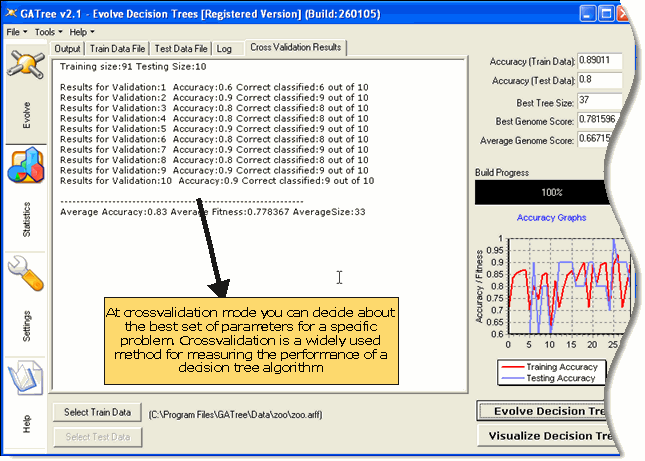Figure 6: Cross-Validation

Case Study

In this small example we will illustrate the effect of different settings on the evolution process, as well as, how to interpret graphs and results.

We start by selecting a training data set and a testing data set (Figure 7). The testing data set is used to evaluate the performance of our model. Make sure that the training and testing data set use the same data format and their data do not overlap.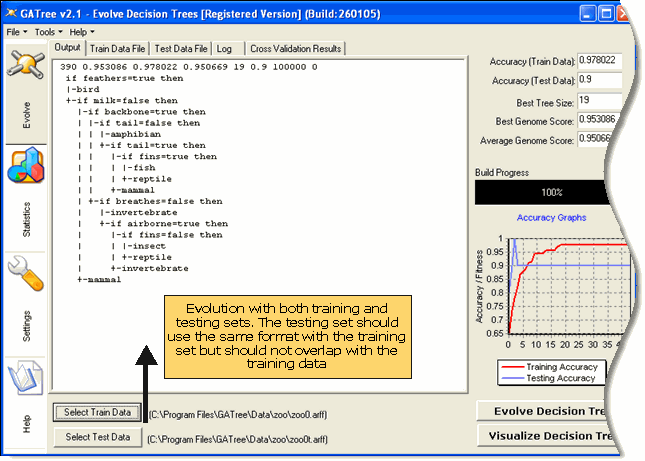Figure 7: Selecting a training and a testing data set

GATree uses ARFF as its standard source format (Figure 8). An ARFF file is a simple text file that describes the problem instances and its attributes. For more info on ARFF format try this url (Search for ARFF Format)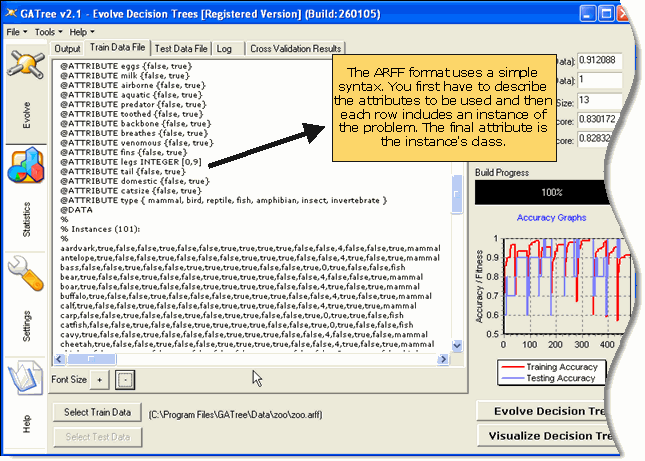Figure 8: The “zoo” dataset at .ARFF Format

The statistics tab provides useful inside on the evolution process (Figure 9). The training and testing accuracy should increase through time. Also, tree size should decrease at the final steps of the evolution, where the accuracy stabilize and there is more room for tree structure improvements.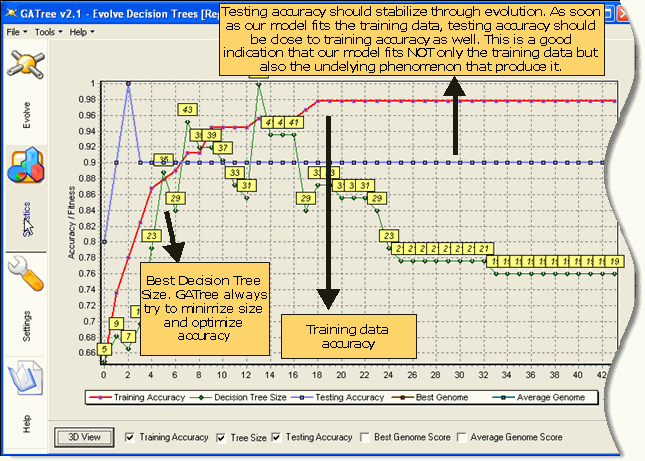Figure 9: Evolution graphs through time

An interesting characteristic of GATree is that we can control its bias towards more accurate or smaller decision trees. To illustrate this effect we go to settings tab and we slide the trackbar in order to prefer very small decision trees (Figure 10)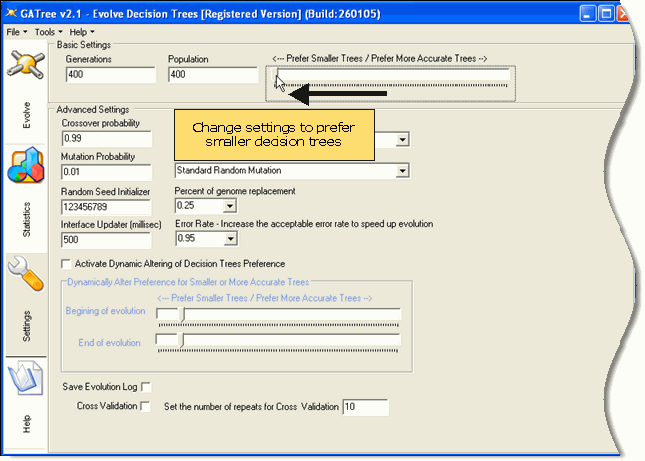Figure 10: Altering settings to bias evolution towards smaller decision trees

The evolution process with the new settings produces a much smaller decision tree (Figure 11)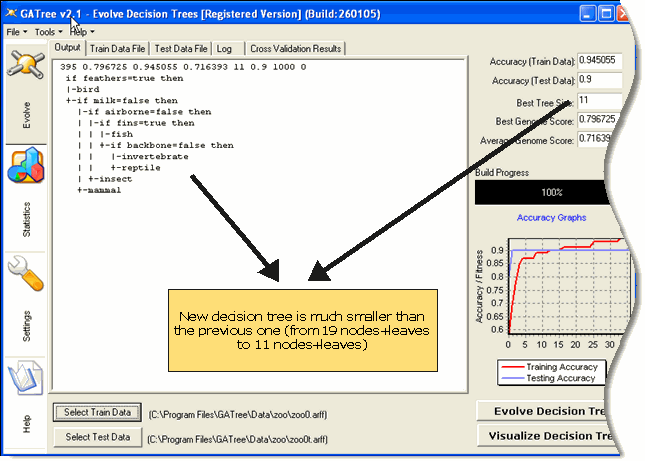Figure 11: A decision tree that is biased towards smaller size

The statistics tab gives us an overview of how the new decision tree fits training and testing data (Figure 12). The new decision tree fits equally well the testing data but not as good the training data. However, from just one experiment we cannot be sure which decision tree is the best for the underlying model.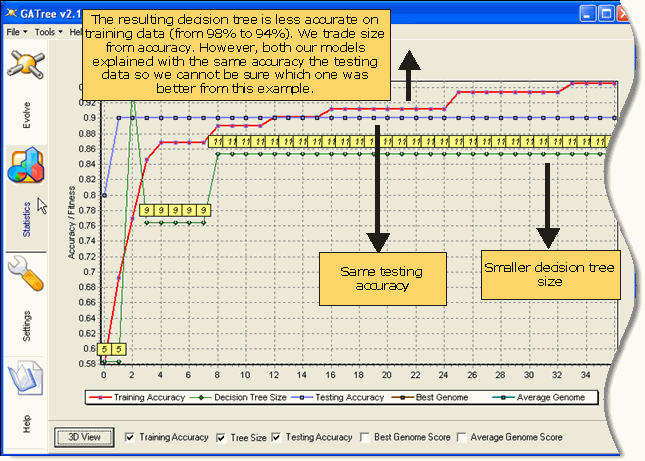Figure 12: Evolution graphs for the altered settings

A much better way to check the quality of the output, under specific settings, is through a cross-validation experiment. You can enable this mode from settings tab as illustrated at Figure 13.Figure 13: Enabling cross validation mode

When cross validation is enabled the program will use only the training data set. It will internally shuffle and partition the data to as needed buckets and perform evolution to all of them. Figure 14 shows the cross validation results for the small tree bias and Figure 15 shows the cross validation results for the big tree bias. From the results it is clear that for that specific problem the small bias produces less accurate but a lot smaller decision trees.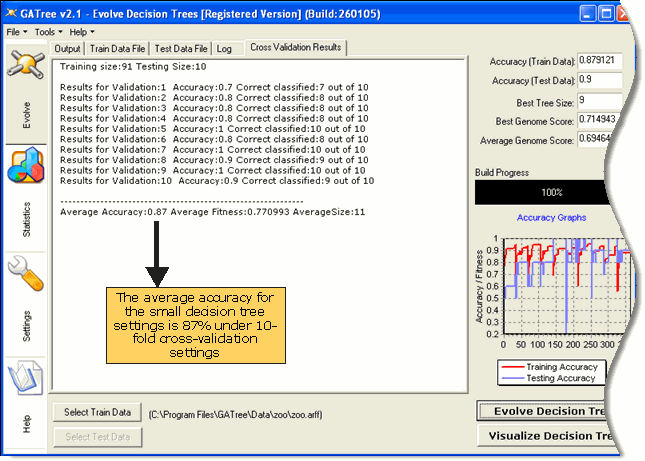Figure 14: Cross validation for small trees bias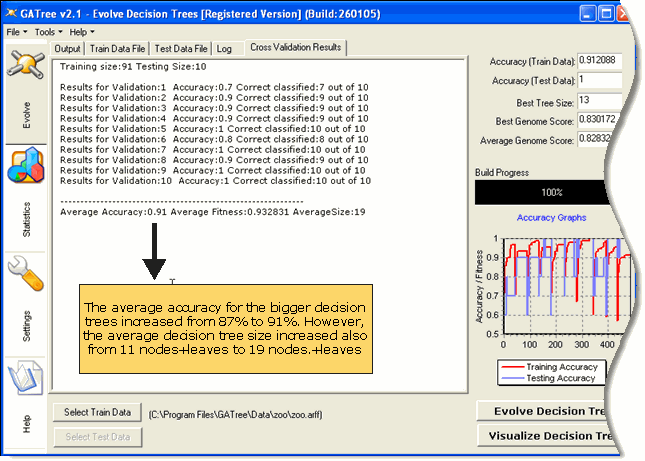Figure 15: Cross validation for big trees bias